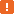# Utilities.FloatToStrF MethodThis method is obsolete. See the Remarks section below.

### Description

The `Utilities.FloatToStrF` method converts a floating-point value to a string.

### Declaration

Utilities.FloatToStrF(Value, Format, Precision, Digits)

 Value [in] Required Double Format [in] Required Integer Precision [in] Required Integer Digits [in] Required Integer Result String

### Applies To

The method is applied to the following object:

### Parameters

The method has the following parameters:

Value

Specifies the floating-point value to be converted to a string.

Format

Specifies the format settings to be used for conversion. Format can be one of the following constants:

Constant Value Description
ffGeneral 0 General format. The function converts the Value to the shortest possible string. Trailing zeros are removed and the decimal point appears only if it is necessary. The resulting string has the scientific format unless the number of digits to the left of the decimal point in the Value is less than or equal to the specified Precision, or unless the Value is greater than or equal to 0.00001. In these cases, the resultant string has the fixed point format. If the scientific format is used, the Digits parameter specifies the minimum number of digits in the exponent (from 0 to 4).
ffExponent 1 Scientific format. The Value is converted to a string like d.ddE+dd. This format implies that there is one digit before the decimal point. The total number of digits in the resultant string is specified by the Precision parameter. The exponent may have up to four digits and always has the sign (+ or -). The Digits parameters specifies the minimum number of digits in the exponent (from 0 to 4).
ffFixed 2 Fixed point format. The Value is converted to string like dd.dddd. There can be one or more digits before the decimal point. The number of digits after the decimal point is specified by the Digits parameter. If this number is greater than the Precision, the resultant string will have the scientific format.
ffNumber 3 Number format. This format is similar to the fixed point format (ffFixed) except that the resulting string includes thousand separators.
ffCurrency 4 Currency format. Converts the Value to a string representing a currency value. For conversion, the `FloatToStrF` method uses the `CurrencyString`, `CurrencyFormat`, `NegCurrFormat`, `ThousandSeparator` and `DecimalSeparator` variables provided by the Utilities plugin. The values of these variables depend on the Regional Settings in the Control Panel on your computer. The Digits parameter specifies the number of digits after the decimal point.

Precision

Specifies the value precision. It should be 15 or less.

Digits

Specifies the format settings to be used for conversion.

### Result Value

If the Value is positive infinity, the function returns INF. If the Value is negative infinity, the function returns -INF.

### Remarks

Regardless of the selected format, the characters used as decimal and thousand separators are specified by the `DecimalSeparator` and `ThousandSeparator` properties.

This method is obsolete. It is supported for backward compatibility only. To convert a floating-point value to a string using a specific format, use the `aqString.Format` method.